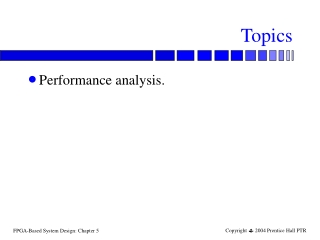DownloadDownload PresentationTopics

# Topics

Download Presentation## Topics

- - - - - - - - - - - - - - - - - - - - - - - - - - - E N D - - - - - - - - - - - - - - - - - - - - - - - - - - -
##### Presentation Transcript

1. Topics • Performance analysis.

2. Unbalanced delays Logic with unbalanced delays leads to inefficient use of logic: short clock period long clock period

3. Flip-flop-based system performance analysis

4. Flip-flop-based system model • Clock signal is perfect (no rise/fall), period P. • Clock event on rising edge. • Setup time s. • Time from arrival of combinational logic event to clock event. • Propagation time p. • Time for value to go from flip-flop input to output. • Worst-case combinational delay C. • Time from output of flip-flop to input.

5. Clock parameters

6. Clock period constraint p • P >= C + s + p. s C

7. Clock with rise/fall

8. Rise/fall clock period constraint p • P >= C + s + p + tr. s tr C

9. Min-max delays • Delays may vary: • Manufacturing variations. • Temperature variations. • Min/max delays compound over paths. • Delays within a chip are correlated. t

10. Latch system clock period • For each phase, phase period must be longer than sum of: • combinational delay; • latch propagation delay. • Phase period depends on longest path.

11. Latch-based system model

12. Two-phase timing parameters

13. Clock period constraint • Total clock period (both phases): • P >= C1 + C2 + 2s + 2p. • Each phase must meet timing for its own latch.

14. Latch-based system model

15. Advanced performance analysis • Latch-based systems always have some idle logic. • Can increase performance by blurring phase boundaries. Results in cycle time closer to average of phases.

16. Example with unbalanced phases One phase is much longer than the other:

17. Spreading out a phase Compute only part of long paths in one phase:

18. Spreading out a phase, cont’d. Use other phase for end of long logic block and all of short logic block:

19. Problems • Hard to debug—can’t stop the system. • Hard to initialize system state. • More sensitive to process variations.

20. D Q Timing and glitches in FSMs • If inputs don’t change, can outputs glitch? logic input output

21. Skew • Skew: relative delay between events. • Signal skew: most important for asynchronous, timing-dependent logic. • Clock skew: can harm any sequential system.

22. Signal skew Machine data signals must obey setup and hold times—avoid signal skew.

23. Signal skew example

24. Clock skew Clock must arrive at all memory elements in time to load data.

25. Clock skew example

26. D D Q Q d Clock skew in system logic

27. Clock skew and qualified clocks

28. s12 = d1 – d2 s21 = d2 – d1 Clock skew analysis model

29. Skew and clock period • Assume that each flip-flop operates instantaneously: • T >= D2 + d12 • If clock arrives at FF2 after FF1, then we have more time to compute. • Given clock period, determine allowable skew: • s12 >= T + D2

30. Timing through logic • As skew increases, we have less time to get the signal through the logic.

31. Clock distribution • Often one of the hardest problems in clock design. • Fast edges. • Minimum skew.

32. D D D D Q Q Q Q Clock skew example 10 ps 10 ps 20 ps 20 ps 30 ps 30 ps

33. Retiming Retiming moves registers through combinational logic:

34. Retiming properties • Retiming changes encoding of values in registers, but proper values can be reconstructed with combinational logic. • Retiming may increase number of registers required. • Retiming must preserve number of registers around a cycle—may not be possible with reconvergent fanout.